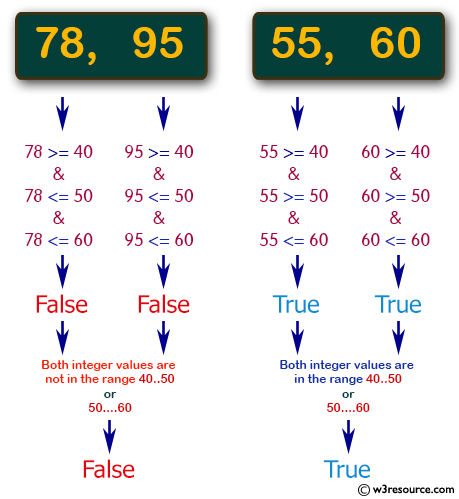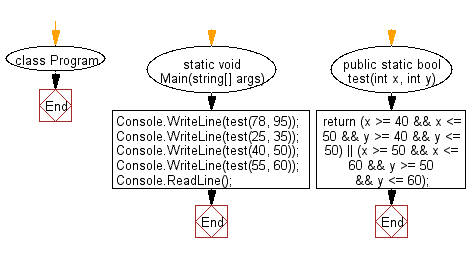﻿ C# - Check two integers both in the range 40..50 or 50..60# C# Sharp Basic Algorithm Exercises: Check whether two given integers are in the range 40..50 inclusive, or they are both in the range 50..60 inclusive

## C# Sharp Basic Algorithm: Exercise-20 with Solution

Write a C# Sharp program to check whether two given integers are in the range 40..50 inclusive, or they are both in the range 50..60 inclusive.

Pictorial Presentation:Sample Solution:

C# Sharp Code:

``````using System;

namespace exercises
{
class Program
{
static void Main(string[] args)
{
Console.WriteLine(test(78, 95));
Console.WriteLine(test(25, 35));
Console.WriteLine(test(40, 50));
Console.WriteLine(test(55, 60));
}

public static bool test(int x, int y)
{
return (x >= 40 && x <= 50 && y >= 40 && y <= 50) || (x >= 50 && x <= 60 && y >= 50 && y <= 60);
}
}
}
```
```

Sample Output:

```False
False
True
True```

Flowchart:C# Sharp Code Editor:

Improve this sample solution and post your code through Disqus

What is the difficulty level of this exercise?

Test your Programming skills with w3resource's quiz.

﻿